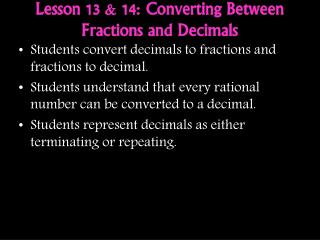DownloadDownload PresentationLesson 13 & 14: Converting Between Fractions and Decimals

# Lesson 13 & 14: Converting Between Fractions and Decimals

Download Presentation## Lesson 13 & 14: Converting Between Fractions and Decimals

- - - - - - - - - - - - - - - - - - - - - - - - - - - E N D - - - - - - - - - - - - - - - - - - - - - - - - - - -
##### Presentation Transcript

1. Lesson 13 & 14: Converting Between Fractions and Decimals • Students convert decimals to fractions and fractions to decimal. • Students understand that every rational number can be converted to a decimal. • Students represent decimals as either terminating or repeating.

2. Numbers in the Real World Places where decimals are used? Places were fractions are used?

3. Example 1: Decimals to Fractions • What is the value of the number 2.25? How can this number be written as a fraction or mixed number? • Rewrite the fraction in simplest form (show your steps). • What is the value of the number 2.025? How can this number be written as a mixed number? • Rewrite the fraction in simplest form (show your steps).

4. Your Turn • Use place value to convert each terminating decimal to a fraction, then rewrite the fraction in its simplest form. • 0.218 c) 2.72 • 0.16 d) 0.0005

5. Example 2: Fractions to Decimals • Write the number as a decimal. Describe your process. Question: How is our denominator related to place value? Goal: Try to obtain a denominator that is on the place value chart! Tenths, hundredths, thousandths, ten thousandths, etc. Write the following numbers as decimals.

6. Your Turn • Convert each fraction to a decimal.

7. But….. • What about… • ‒ We need a plan in case the denominator does not go in to 10, 100 or 1,000…..

8. Vocabulary:Terminating Decimal and Non-Terminating Decimal • Terminating Decimal - A terminating decimal will have a finite number of digits after the decimal point. In other words, it will end. • Non-Terminating Decimal – A non-terminating decimal will not have a finite number of digits after the decimal point. In other words, it will go on forever (it may repeat numbers or it may not repeat numbers).

9. Explore Why does the division bar help us? So if we ever need to find a decimal from a given fraction, we can simply divide! • Using the division button on your calculator, find the decimal equivalents for the following fractions. • Now sort them in to the correct column. Terminating Non-Terminating

10. Fractions to Decimals….By dividing • Use long division to convert the following fractions to decimals. ‒ -